Lecture 7.  Population Genetics V: Effective population size, Ne

The effective population size is the size of an ideal population (i.e., one that meets all the Hardy-Weinberg assumptions) that would lose heterozygosity at a rate equal to that of the observed population. See Gillespie text pp. 47-49 and Hartl (2000, Eqn 2.47) for how population size affects the decline of heterozygosity.

Because the transmission of genes from one generation to the next is fundamentally a demographic process, the size of the population is an integral part of almost any population genetics analysis. Put differently, genetic drift is directly related to population size (small = more drift, large = less drift). The triangle of drift, mutation and migration is the big three for the purposes of understanding a neutral theory approach to population genetic structure.

One doesn't need to look very far through a population genetics text to see N in a population genetics equation. A big problem is that Ne is usually part of a product whose separate terms we may not be able to estimate. For example, we often see the quantity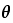(theta):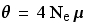Eqn 7.1 where µ (Greek letter mu) is the mutation rate.  An approximate estimator for FST (population structure) is: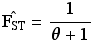Eqn 7.2 Since we can often estimate FST by other means (as we saw using the observed and expected heterozygosity method) we actually often rearrange this to get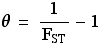Eqn 7.3 If were lucky enough to have either a good estimate of effective population size (from a demographic angle) or of the mutation rate (µ), then we can estimate the two components. Usually, however, we dont. If we want to compare relative population size over the same loci, however, we can do that (as well see when I get to the bear data). The (reasonable-seeming) assumption here is that the mutation rate will be the same over the same loci and closely related populations. A maximum likelihood program by R. Nielsen (1997; Misat.1 http://www.biom.cornell.edu/Homepages/Rasmus_Nielsen/misat/misat.1.0.exe) allows an estimate offrom microsatellite data.

What factors affect Ne? In general, Ne is less than the census population size N (the actual number of animals present). In some cases it is far lower. We will examine five forces acting to make Ne (effective population size) differ from N (census population size, or number of animals actually present).

1) One of the most important influences reducing Ne relative to N is fluctuating population size. This is because Ne that accounts for fluctuating population size is calculated as the harmonic mean of the census size. The harmonic mean is the reciprocal of [the average of the reciprocals]. An example will clarify both the meaning of this and its dramatic impact. Say we have a population census over four time periods of 200, 150, 50, and 300. What is the estimate of Ne?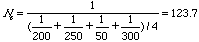Eqn 7.4 The harmonic mean of 123.7 contrasts with the arithmetic mean of 200. See the Gillespie texts derivation on pp. 47-49. Another relevant paper is by Vucetich et al. (1997).

2) A second factor affecting Ne is the breeding sex ratio. A famous equation for dairy cows shows the dramatic effect a very skewed sex ratio can have. Say we have 96 cows and 4 bulls as the "breeding herd". What is Ne? The equation is:Eqn 7.5 The sex ratio effective size of 15.4 is much closer to the number of bulls than to the number of cows. See Gillespie text example on p. 49. Try putting 50:50 in Eqn 7. 5 to verify that Ne = N when the sex ratio is 1:1.  [The sex ratio effect I have described is a special case of a more general impact on effective size due to variance in reproductive success].

3) A third influence on Ne can have an interesting effect that sometimes enters into captive breeding designs. Ne assumes a Poisson distribution of family (offspring) numbers. The Poisson is characterized by having the variance equal the mean. If the variance is lower than the mean, then Ne can actually be larger than the census size! Zoos will sometimes maintain their captive breeding stock to equalize family sizes (zero variance). This can increase Ne. They will usually need to keep "reserve" breeders, in case of the death of one of the selected breeders. In natural populations, if the environment causes the variance to exceed the mean (which may occur fairly frequently) then Ne will again be less than N. Hartl (2000, p. 96) gives an example of the reduction of Ne relative to N because of variance in family size.

4) Overlapping generations can also act to reduce Ne. (Felsenstein, 1971).

5) Yet another factor affecting Ne is the spatial dispersion (pattern of spatial distribution) of the population. Its influence on the effective size is given by: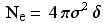Eqn 7.6 whereis the variance of the dispersal distance and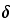is the density of individuals. This formulation is often called the neighborhood size and assumes a normal (bell-shaped) distribution of dispersal distances (out in a circular shape from the source, hence the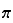). So, again, changes in the variance of dispersal size can affect Ne (viscous populations will have smaller Ne). Note that the units of density are units of area (e.g., hectares) and that the variance will be distance squared (= area), so the distance units will cancel, giving us a "pure number" of individuals, which is what we should expect. Heres a place where good natural history and demographic data might produce reasonably good estimates of Ne. Hartl (2000, p. 98) gives an example of calculating Ne using this approach. Woolfenden and Fitzpatrick (1984) used this approach to estimate Ne for Florida Scrub-Jays -- their estimate of Ne was 298.

A major problem, though, is how to account simultaneously for all these various effects -- the jury is definitely still out on that one. Technical discussions of Ne include important papers by Caballero (1994), Crow and Denniston (1988), Harris and Allendorf (1989), various papers by Nunney, Vucetich et al. (1997), and Whitlock and Barton (1997).  Waples (1989) showed how to estimate Ne by using temporal fluctuations in allele frequencies.  Wang and Whitlock (2003) incorporated variation in both time and space as bases for estimating Ne.

In the literature, you may see "variance effective size" and "inbreeding effective size".  The former focuses on changes in genetic variance, on consequences for the offspring generation and hence naturally leads to consideration of interpopulation divergence.  The latter focuses on changes in heterozygosity, on consequences for the parental generation, and hence naturally leads to consideration of the level of inbreeding within populations.  See Crow and Kimura's (1970) text (pp. 345-364) for a much more detailed treatment of the different derivations and distinctions.

References:

Caballero, A. 1994. Review article: Developments in the prediction of effective population size. Heredity 73: 657-679.

Chesser, R.K., and R.J. Baker. 1996. Effective sizes and dynamics of uniparentally and diparentally inherited genes. Genetics 144: 1225-1235.

Crow, J.F., and C. Denniston. 1988. Inbreeding and variance effective population effective numbers. Evol. 42: 482-495.

Crow, J.F., and M. Kimura. 1970. An Introduction to Population Genetics Theory. Burgess Publishing, Minneapolis, MN.

Felsenstein, J. 1971. Inbreeding and variance effective numbers in populations with overlapping generations. Genetics 68: 581-597.

Harris, R.B., and F.W. Allendorf. 1989. Genetically effective population size of large mammals: an assessment of estimators. Conserv. Biol. 3: 181-191.

Hartl, D.L. 2000. A Primer of Population Genetics (3rd ed.). Sinauer Associates, Sunderland, MA

Hedrick, P.W., and M.E. Gilpin. 1997. Genetic effective size of a metapopulation. Pp. 165-181 In Metapopulation Biology: Ecology, Genetics and Evolution (I. Hanski, and M.E. Gilpin, eds.). Academic Press, NY.

Nielsen, Rasmus. 1997. A likelihood approach to populations samples of microsatellite alleles. Genetics 146: 711-716

Nunney, L. 1995. Measuring the ratio of effective population size to adult numbers using genetic and ecological data. Evolution 49: 389-392.

Nunney, L. 1999. The effective size of a hierarchically structured population. Evol. 53: 1-10.

Nunney, L., and K.A. Campbell. 1993. Assessing minimum viable population size: demography meets population genetics. Trends Ecol. Evol. 8: 234-239.

Vucetich, J.A., T.A. Waite, and L. Nunney. 1997. Fluctuating population size and the ratio of effective to census population size. Evol. 51: 2017-2021.

Wang, J., and M.C. Whitlock. 2003. Estimating effective population size and migration rates from genetic samples over space and time. Genetics 163: 429-446.

Waples, R.S. 1989. A generalized method for estimating effective population size from temporal changes in allele frequency. Genetics 121: 379-391.

Whitlock, M.C., and N.H. Barton. 1997. The effective size of a subdivided population. Genetics 146: 427-441.

Woolfenden, G.E. and J.W. Fitzpatrick. 1984. The Florida Scrub Jay: Demography of a Cooperative-breeding Bird. Monogr. Pop. Biol. 20. Princeton Univ. Press, Princeton, N.J.